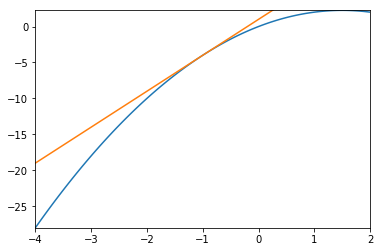# Truncation Error vs Rounding Error¶

In this notebook, we'll investigate two common sources of error: Truncation error and rounding error.

In :
import numpy as np
import matplotlib.pyplot as pt


Task: Approximate a function (here: a parabola, by a line)

In :
center = -1
width = 6

def f(x):
return - x**2 + 3*x

def df(x):
return -2*x + 3

grid = np.linspace(center-width/2, center+width/2, 100)

fx = f(grid)
pt.plot(grid, fx)
pt.plot(grid, f(center) + df(center) * (grid-center))

pt.xlim([grid, grid[-1]])
pt.ylim([np.min(fx), np.max(fx)])

Out:
(-28.0, 2.2497704315886136)• What's the error we see?
• What if we make width smaller?